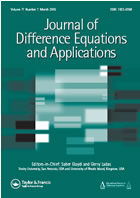# Research

A picture is worth a thousand words. So, here is the “mathematical picture” of objects that I am interested in
$$\int_0^{\infty}\left(\frac{2}{e^t+e^{-t}}\right)^me^{-st}dt= \displaystyle{\frac{1}{s+\displaystyle{\frac{m}{s+\displaystyle{\frac{2(m+1)}{s+\displaystyle{\frac{3(m+2)}{s+\ddots}}}}}}}}, \quad m\ge 0,\quad s>0$$
or, even a better one, for Apéry’s constant we have that
$$\zeta(3)=\sum_{n=1}^{\infty}\frac{1}{n^3}}= 1+\displaystyle{\frac{1/4}{1+\displaystyle{\frac{1/3}{1+\displaystyle{\frac{2/3}{1+\displaystyle{\frac{6/5}{1+\ddots}}}}}}}}$$
Both of these continued fractions were derived by Stieltjes more than a century ago but such expansions and relations keep appearing here and there up to these days. They also take different faces and more sophisticated forms, which is quite amazing. Now, I am in the position to give an idea about my research interests in just one sentence: generally speaking, one of the main goals is to find characterizations of the entries of continued fractions (the right-hand side) in terms of the properties of what we have on the left and vice versa. Regarding the first formula, I should say that, in modern mathematics, such problems are rather formulated in terms of difference operators (so-called Jacobi matrices, their generalizations, and various analogs) than in terms of continued fractions. By the way, approximants to continued fractions usually give “good” approximations, which is extensively exploited in numerical mathematics.

If you want to know more about my research, reviews of my papers can be found on MathSciNet or on zbMATH.. You will need a subscription to access those websites. Alternatively, my Google Scholar profile is here and it can give links to published papers as well as to preprints. The publications tab of this webpage contains links to the preprints available on ArXiv.Org.

I am on the Editorial Board of Journal of Difference Equations and Applications. If you want to submit your paper to the journal, click here.